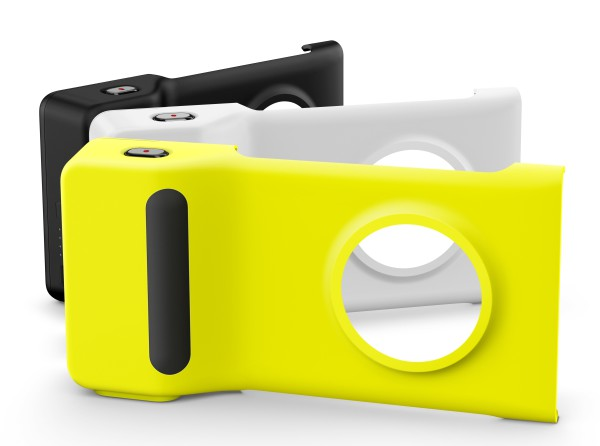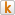Názory zde publikované mohou a nemusí reprezentovat názory mého zaměstnavatele.

# kmb02ProEXR File Description

=Attributes=
cameraAperture (float): 36.000004
cameraFNumber (float): 5.000000
cameraFarClip (float): 4000.000000
cameraFarRange (float): 999999984306749440.000000
cameraFocalLength (float): 8.333280
cameraFov (float): 39.599079
cameraNearClip (float): 10.000000
cameraNearRange (float): 0.000000
cameraProjection (int): 0
cameraTargetDistance (float): 1.000000
cameraTransform (m44f)
channels (chlist)
compression (compression): Zip16
dataWindow (box2i): [0, 0, 6999, 6999]
displayWindow (box2i): [0, 0, 6999, 6999]
lineOrder (lineOrder): Increasing Y
pixelAspectRatio (float): 1.000000
screenWindowCenter (v2f): [0.000000, 0.000000]
screenWindowWidth (float): 1.000000

=Channels=
A (half)
AO_VRayDirt1.B (half)
AO_VRayDirt1.G (half)
AO_VRayDirt1.R (half)
B (half)
BACK.B (half)
BACK.G (half)
BACK.R (half)
FILL.B (half)
FILL.G (half)
FILL.R (half)
G (half)
KEY.B (half)
KEY.G (half)
KEY.R (half)
R (half)
Z (half)
bumpnormals.X (half)
bumpnormals.Y (half)
bumpnormals.Z (half)
diffuse.B (half)
diffuse.G (half)
diffuse.R (half)
lighting.B (half)
lighting.G (half)
lighting.R (half)
normals.X (half)
normals.Y (half)
normals.Z (half)
rawReflection.B (half)
rawReflection.G (half)
rawReflection.R (half)
rawRefraction.B (half)
rawRefraction.G (half)
rawRefraction.R (half)
reflect.B (half)
reflect.G (half)
reflect.R (half)
refract.B (half)
refract.G (half)
refract.R (half)
specular.B (half)
specular.G (half)
specular.R (half)Send to Kindle